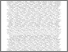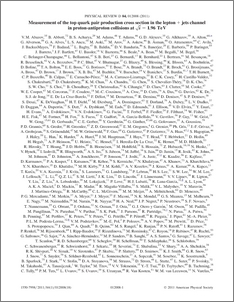# Measurement of the top quark pair production cross section in the lepton+jets channel in proton-antiproton collisions at $\sqrt{s}$=1.96 TeV

Collaboration, D0 and Bertram, Iain and Borissov, Guennadi and Fox, Harald and Ross, Anthony and Williams, Mark and Ratoff, Peter (2011) Measurement of the top quark pair production cross section in the lepton+jets channel in proton-antiproton collisions at $\sqrt{s}$=1.96 TeV. Physical Review D, 84 (1). ISSN 1550-7998Preview
PDF
PhysRevD.84.012008.pdf - Published Version

## Abstract

We present a measurement of the inclusive top quark pair production cross section in \ppbar collisions at (\sqrt{s}=1.96) TeV utilizing data corresponding to an integrated luminosity of \lumi\ collected with the D0 detector at the Fermilab Tevatron Collider. We consider final states containing one high-$p_{T}$ isolated electron or muon and at least two jets, and we perform three analyses: one exploiting specific kinematic features of \ttbar events, the second using $b$-jet identification, and the third using both techniques to separate \ttbar\ signal from background. In the third case, we determine simultaneously the $t\bar{t}$ cross section and the ratio of the production rates of $W$+heavy flavor jets and $W$+light flavor jets, which reduces the impact of the systematic uncertainties related to the background estimation. Assuming a top quark mass of 172.5 GeV, we obtain $\sigma_{t\bar{t}} = 7.78^{+0.77}_{-0.64}$ pb. This result agrees with predictions of the standard model.

Item Type:
Journal Article
Journal or Publication Title:
Physical Review D